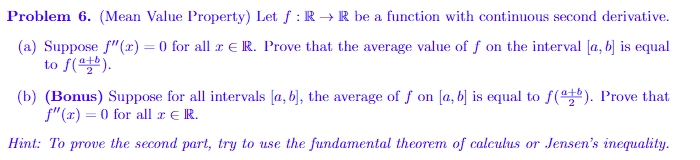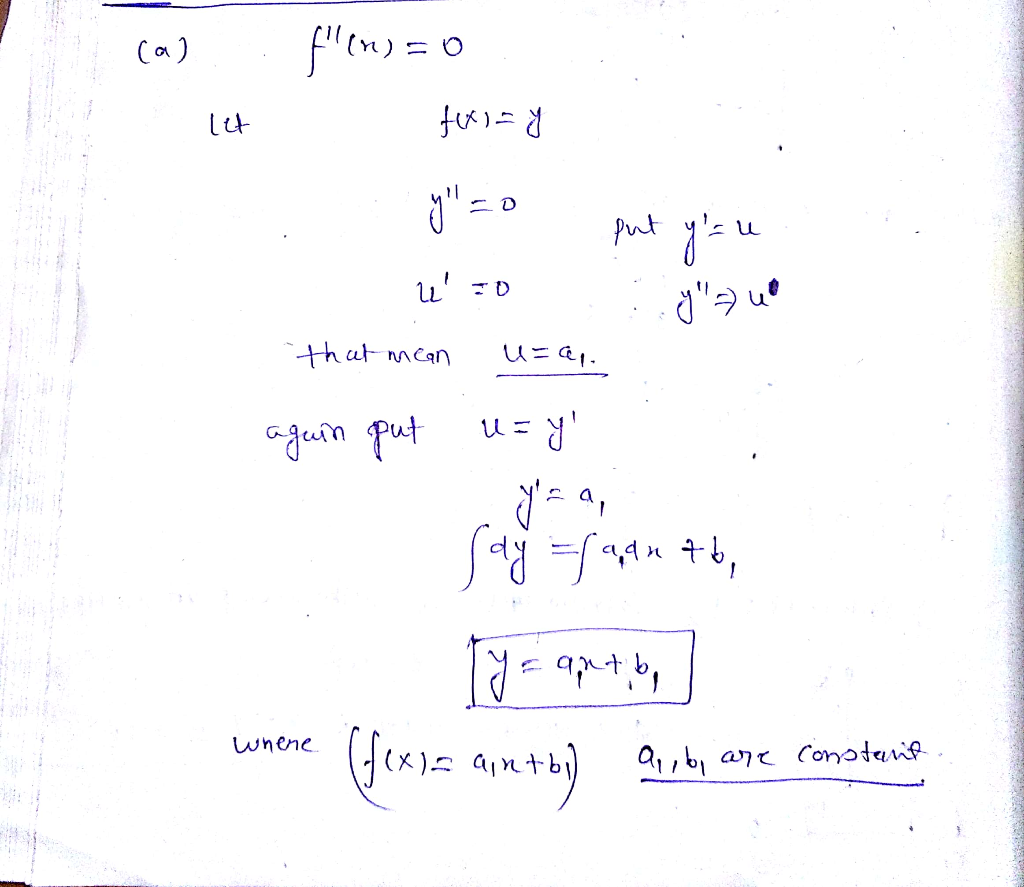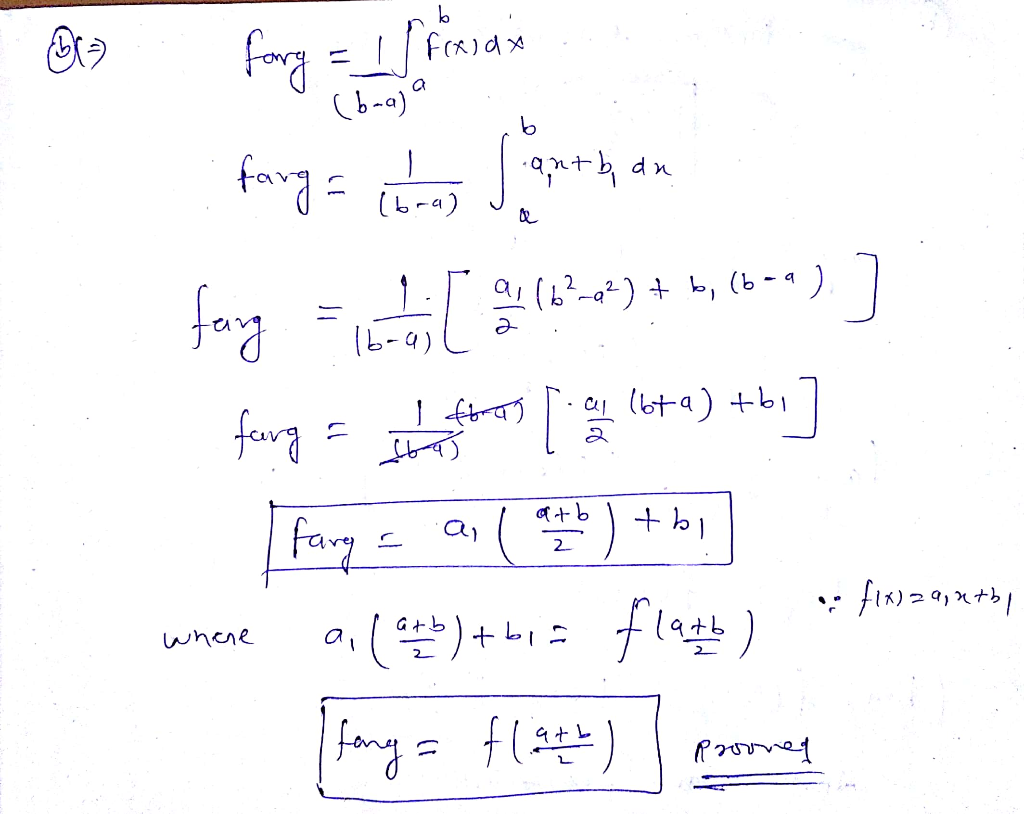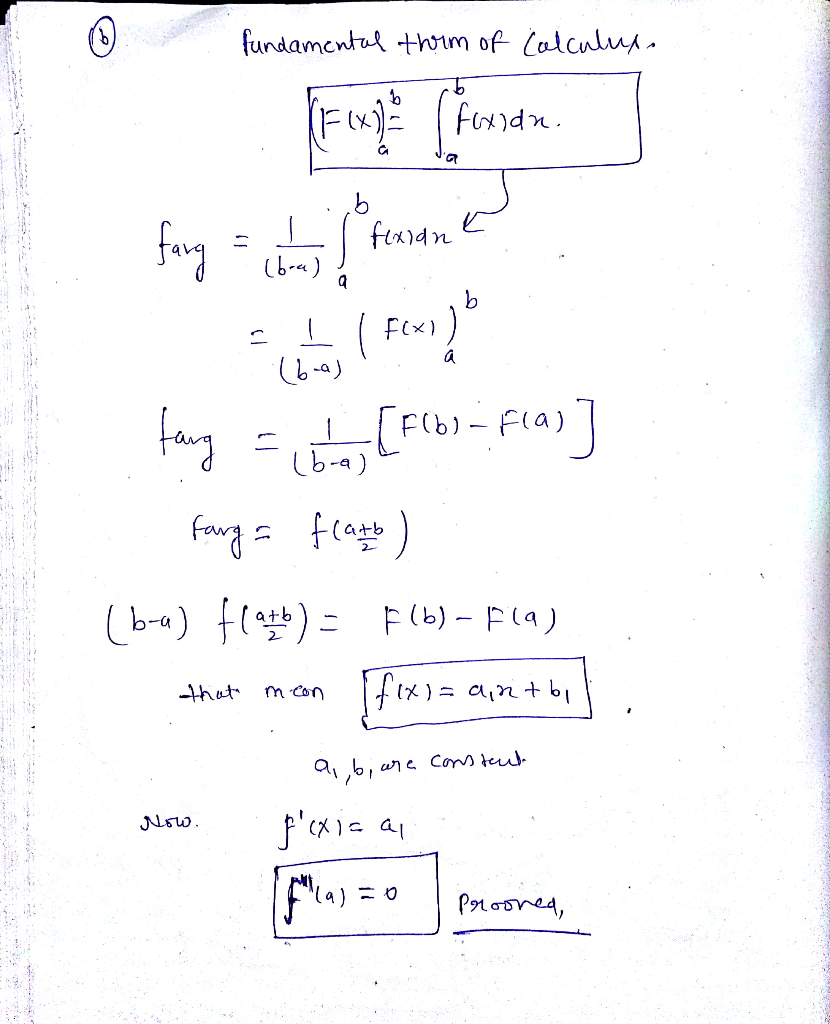# Problem 6. (Mean Value Property) Let f : RR be a function with continuous second derivative. (a) Suppose f"( to f(...Problem 6. (Mean Value Property) Let f : RR be a function with continuous second derivative. (a) Suppose f"( to f( ). 0 for all r E IR. P al rove that the average value of f on the interval a, bs equ f, onla b is equal tore !) Prove intervals la, b, the average。 (b) (Braus) Supposeerall Hint: To prove the second part, try to use the fundamental theorem of calculus or Jensen's inequality.##### Add Answer of: Problem 6. (Mean Value Property) Let f : RR be a function with continuous second derivative. (a) Suppose f"( to f(...
More Homework Help Questions Additional questions in this topic.